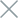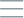• Home
• Tags
• Series
•# Comprehensions in Python: List, Dictionary, Set

## List Comprehensions

• List comprehensions are a concise and efficient way to create lists in Python.
• They allow you to generate a new list by applying an expression to each item in an existing iterable (e.g., list, tuple, string) and filtering the items based on a condition.
• The syntax of a list comprehension is: `[expression for item in iterable if condition]`

Example:

``````# List comprehension to generate a list of squares of numbers from 0 to 9
squares = [x**2 for x in range(10)]
print(squares)   # Output: [0, 1, 4, 9, 16, 25, 36, 49, 64, 81]

# List comprehension to filter even numbers from a list
numbers = [1, 2, 3, 4, 5, 6, 7, 8, 9, 10]
even_numbers = [x for x in numbers if x % 2 == 0]
print(even_numbers)   # Output: [2, 4, 6, 8, 10]
``````

## Dictionary Comprehensions

• Dictionary comprehensions allow you to create dictionaries in a concise manner.
• Similar to list comprehensions, they generate a new dictionary by applying an expression to each item in an iterable and filtering items based on a condition.
• The syntax of a dictionary comprehension is: `{key_expression: value_expression for item in iterable if condition}`

Example:

``````# Dictionary comprehension to create a dictionary of squares of numbers from 0 to 9
squares_dict = {x: x**2 for x in range(10)}
print(squares_dict)   # Output: {0: 0, 1: 1, 2: 4, 3: 9, 4: 16, 5: 25, 6: 36, 7: 49, 8: 64, 9: 81}

# Dictionary comprehension to filter even numbers from a dictionary
numbers_dict = {1: 'one', 2: 'two', 3: 'three', 4: 'four', 5: 'five'}
even_numbers_dict = {key: value for key, value in numbers_dict.items() if key % 2 == 0}
print(even_numbers_dict)   # Output: {2: 'two', 4: 'four'}
``````

## Set Comprehensions

• Set comprehensions allow you to create sets in a similar way to list comprehensions and dictionary comprehensions.
• They generate a new set by applying an expression to each item in an iterable and filtering items based on a condition.
• The syntax of a set comprehension is: `{expression for item in iterable if condition}`

Example:

``````# Set comprehension to create a set of squares of numbers from 0 to 9
squares_set = {x**2 for x in range(10)}
print(squares_set)   # Output: {0, 1, 4, 9, 16, 25, 36, 49, 64, 81}

# Set comprehension to filter even numbers from a set
numbers_set = {1, 2, 3, 4, 5, 6, 7, 8, 9, 10}
even_numbers_set = {x for x in numbers_set if x % 2 == 0}
print(even_numbers_set)   # Output: {2, 4, 6, 8, 10}
``````

Comprehensions in Python provide a concise and readable way to create lists, dictionaries, and sets based on existing iterables, making your code more elegant and efficient.